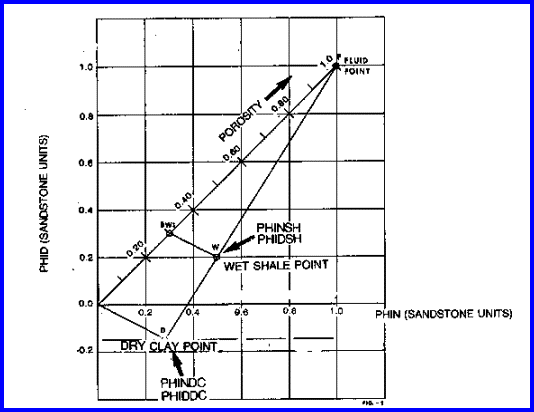Bulk Volume Water (Dual Water) Model
The bulk volume water solution is an alternate density neutron method which partitions the water in the formation into the free water in the pores and the bound water in the shale. Water saturation is obtained from the ratio of free water to effective pore volume.

This is another version of the Shaly Sand Models and suffers all the same problems as other shaly sand models, particularly the problem of heavy minerals. Go HERE to read the warnings and cures.

A superior model that avoids these problems is the Shale Corrected Density Neutron Complex Lithology Crossplot Method. The Meta/Kwik spreadsheet for this model is available atBulk Volume Water (Dual Water)
A major assumption required is the value of the apparent density log porosity of dry clay in the shale matrix (PHIDDC). This is often a negative number because the dry clay is more dense (lower porosity) than pure quartz.

Calculate neutron log dry clay point from density dry clay value,
1: PHINDC = 1.00 - (1.00 - PHIDDC ) * (1.00 - PHINSH) / (1.00 - PHIDSH)

Calculate bulk volume of bound water in shale.
2: BVWSH = (PHINDC * PHIDSH - PHIDDC * PHINSH) / (PHINDC - PHIDDC)

Calculate total porosity, also called total bulk volume of water.
3: PHIt = (PHINDC * PHID - PHIDDC * PHIN) / (PHINDC - PHIDDC)

Calculate effective porosity.
4: PHIbvw = PHIt - Vsh * BVWSH

Where:
BVWSH = bulk volume of water attached to 100% shale (fractional)
PHIbvw = effective porosity from BVW method (fractional)
PHID = density log reading (fractional)
PHIDDC = density dry clay point (fractional)
PHIDSH = density shale point (fractional)
PHIN = neutron log reading (fractional)
PHINDC = neutron dry clay point (fractional)
PHINSH = neutron shale point (fractional)
PHIt = total porosity (fractional)
Vsh = shale volume (fractional)COMMENTS:
The formulae are shown graphically in below.Chart for Dual Water Porosity Model - note that Dry Clay has a negative density porosity.  The dry clay point is found from an extrapolation from the water point through the wet shale point down to the dry clay density (which is based on the clay mineral present in the shaly sand).

This method is mathematically similar to the shaly sand crossplot method. If matrix offset is required for heavy minerals, apply the offset to all neutron and density values including shale points first, then use the above equation. The method is also called the dual water method and is the basis of many wellsite and office computer programs.

Nothing special is done in gas zones, as the values computed for PHIt and PHIe are reasonable even if gas crossover occurs.RECOMMENDED PARAMETERS:
The usual value for PHIDDC is in the range of minus 0.11 to minus 0.15. It cannot be picked by observation of logs or crossplots. It can be calculated from:
5: PHIDDC = (DENSDC - DENSW) / (DENSMA - DENSW).NUMERICAL EXAMPLE:
1. Assume data for Sand "D".
PHID = 0.12
PHIN = 0.28
DENSMA = 2650 kg/m3 (no offset)
PHIDSH = 0.03
PHINSH = 0.30

Vsh = (0.28 - 0.12 ) / (0.30 – 0.03) = 0.59
Select PHIDDC = - 0.13 by calculating dry clay porosity from dry clay density,
PHINDC = 1.00 - (1.00 - (- 0.13)) * (1.00 - 0.30) / (1.00 - 0.03) = 0.184
BVWSH = (0.184 * 0.03 - (-0.13) * 0.30) / (0.184 - (-0.13)) = 0.142
PHIt = (0.184 * 0.12 - (-0.13) * 0.28) / (0.184 - (-0.13)) = 0.186
PHIbvw = 0.186 - 0.59 * 0.14 = 0.103

Page Views ---- Since 01 Jan 2015
Copyright 2023 by Accessible Petrophysics Ltd.
CPH Logo, "CPH", "CPH Gold Member", "CPH Platinum Member", "Crain's Rules", "Meta/Log", "Computer-Ready-Math", "Petro/Fusion Scripts" are Trademarks of the Author